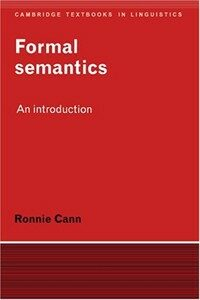> Detail View

Detail ViewFormal semantics: an introduction (Loan 27 times)

Material type
단행본
Personal Author
Cann, Ronnie.
Title Statement
Formal semantics: an introduction / Ronnie Cann.
Publication, Distribution, etc
Cambridge ;   New York, NY, USA :   Cambridge University Press ,   1993.
Physical Medium
xvii, 344 p. : ill. ; 24 cm.
Series Statement
Cambridge textbooks in linguistics
ISBN
0521374634 (hardback) 0521376106 (pbk.)
Bibliography, Etc. Note
Includes bibliographical references (p. 333-338) and index.
Semantics. Semantics (Philosophy)
 000 00873camuu2200265 a 4500 001 000045164752 005 20050426111301 008 920309s1993 enka b 00 0 eng 010 ▼a 92008538 020 ▼a 0521374634 (hardback) 020 ▼a 0521376106 (pbk.) 035 ▼a KRIC00297457 040 ▼a 211032 ▼c 211032 ▼d 211009 050 0 0 ▼a P325 ▼b .C28 1993 082 0 0 ▼a 401/.43 ▼2 20 090 ▼a 401.43 ▼b C224f 100 1 ▼a Cann, Ronnie. 245 1 0 ▼a Formal semantics: ▼b an introduction / ▼c Ronnie Cann. 260 ▼a Cambridge ; ▼a New York, NY, USA : ▼b Cambridge University Press , ▼c 1993. 300 ▼a xvii, 344 p. : ▼b ill. ; ▼c 24 cm. 490 0 ▼a Cambridge textbooks in linguistics 504 ▼a Includes bibliographical references (p. 333-338) and index. 650 0 ▼a Semantics. 650 0 ▼a Semantics (Philosophy)

Holdings Information

No. Location Call Number Accession No. Availability Due Date Make a Reservation Service
No. 1 Location Call Number 401.43 C224f Accession No. 111318110 Availability Available Due Date Make a Reservation Service
No. 2 Location Call Number 401.43 C224f Accession No. 412912481 Availability Available Due Date Make a Reservation Service

Contents information

CONTENTS
List of figures page = xii
Preface = xiii
List of symbols = xv
1 INTRODUCTION = 1
1.1 Semantics and semantic theory = 1
1.1.1 Compositionality = 2
1.1.2 Meaning relations = 5
1.1.3 Amibiguity = 8
1.1.4 Denotation = 10
1.2 Interpretation and representation= 13
1.2.1 Truth-conditions = 15
1.2.2 Formatisation, models and sets = 17
1.3 Beyond truth-conditions = 19
1.3.1 Knowledge, behaviour and use = 19
1.3.2 Context = 22
1.4 A note on method = 23
2 PREDICATES AND ARGUMENTS = 27
2.1 Translating English into a logical language = 27
2.1.1 The syntax of Lp = 27
2.1.2 A grammar fragment for English = 28
2.1.3 The translation procedure = 32
2.2 Interpreting Lp = 38
2.2.1 Individuals and identity = 41
2.2.2 A little light set theory = 44
2.2.3 Interpreting predicates = 47
2.2.4 Finishing Up = 52
3 NEGATION AND CO-ORDINATION = 54
3.1 Compound sentences = 54
3.2 Complex formulae = 55
3.3 Interpretation = 60
3.3.1 Negation = 60
3.3.2 Conjunction = 63
3.3.3 Disjunction = 67
3.3.4 Implication = 70
3.3.5 Equivalence = 73
3.4 Formal interpretation = 74
3.4.1 Formal models = 75
3.4.2 Model theory = 78
4 TYPE THEORY = 82
4.1 Verb phrases and other constituents = 82
4.2 A typed logical language = 83
4.2.1 Semantic types = 83
4.2.2 Translating verb phrases = 98
4.3 More set theory = 93
4.3.1 Relations and functions = 93
4.3.2 Sets again = 96
4.4 Interpreting Lt ype = 98
4.4.1 Denotation = 99
4.4.2 Revising the theory = 103
5 THE LAMBDA OPERATOR = 112
5.1 The passive = 112
5.2 Introducing the lambda operator = 115
5.2.1 Extending Lt ype = 116
5.2.2 Interpreting lambda expressions = 119
5.2.3 The passive again = 126
5.3 Generalising lambda expressions = 127
5.4 Reviewing co-orditiation = 136
5.4.1 Sentential co-ordination = 136
5.4.2 Co-ordinating other categories = 143
6 QUANTIFICATION = 150
6.1 The variety of noun phrases = 150
6.2 Introducing the logical quantifiers = 151
6.2.1 The quantifiers = 152
6.2.2 Interpreting LQ = 154
6.2.3 Quantification and negation = 158
6.3 A compositional approach = 159
6.3.1 Translating quantifier pronouns = 159
6.3.2 Complex NPs = 162
6.3.3Nominal modifiers = 169
6.4 Proper names and definite descfiptions = 172
6.5 Two problems = 177
6.5.1 Type raising = 177
6.5.2 Scope ambiguities = 180
6.6 Gencralised quantifiers = 187
7 INFERENCE = 197
7.1 Making inferences = 197
7.2 Logical deduction = 200
7.2 1 Using the connectives = 204
7.2.2 Reasoning with quantifiers = 210
7.3 Lexical meaning = 215
7.4 Noii-truth-conditional aspects of the connectives = 224
7.4.1 And = 224
7.4.2 Or = 276
7.4.3 If = 229
8 TIME, TENSE AND ASPECT = 233
8.1 Temporal contingency = 233
8.2 Time = 233
8.2.1 Intervals of time = 234
8.2.2 Temporal models = 236
8.3 Tense = 241
8.3.1 Past, present and future = 241
8.3.2 Complex tenses = 246
8.4 Simple aspect = 251
8.4.1 Perfective and imperfective = 251
8.4.2 States and actions = 256
8.5 Scope ambiguities = 259
9 POSSIBLE WORLDS = 263
9.1 Where entailments fail = 263
9.2 Intension and extension = 267
9.3 Introducing other worlds = 269
9.3.1 Simple modality = 270
9.3.2 Accessible worlds = 276
10 INTENSIONAL SEMANTICS = 282
10.1 Modelling intensions = 282
10.2 The intensional language LI L = 288
10.2.1 Intensional expressions ... = 289
10.2.2 ... and their interpretation = 293
10.3 Interpreting opaque contexts = 299
10.3.1 Oblique transitive verbs = 299
10.3.2 Control verbs = 306
10.3.3 Propositional attitudes = 308
10.4 Two problems = 315
10.4.1 Intensional equivalence = 316
10.4.2 Cross-world reference = 319
10.5 Postscript = 320
Answers to selected exercises = 323
References = 333
Index = 339

New Arrivals Books in Related Fields

파롤

Gusdorf, Georges (2021)

남기춘 (2021)

How languages are learned / 5th ed

Lightbown, Patsy (2021)

Formal semantics : a mathematical approach to the analysis of meaning

Song, Mean-young (2021)

(알아두면 쓸모 있는) 어원잡학사전 : 평범한 단어들의 특별한 어원이야기

Foote, Patrick (2021)

L'intelligence artificielle des textes : des algorithmes à l'interprétation

Mayaffre, Damon (2021)

홍승우 (2021)

The handbook of speech perception / 2nd ed

Pardo, Jennifer S. (2021)